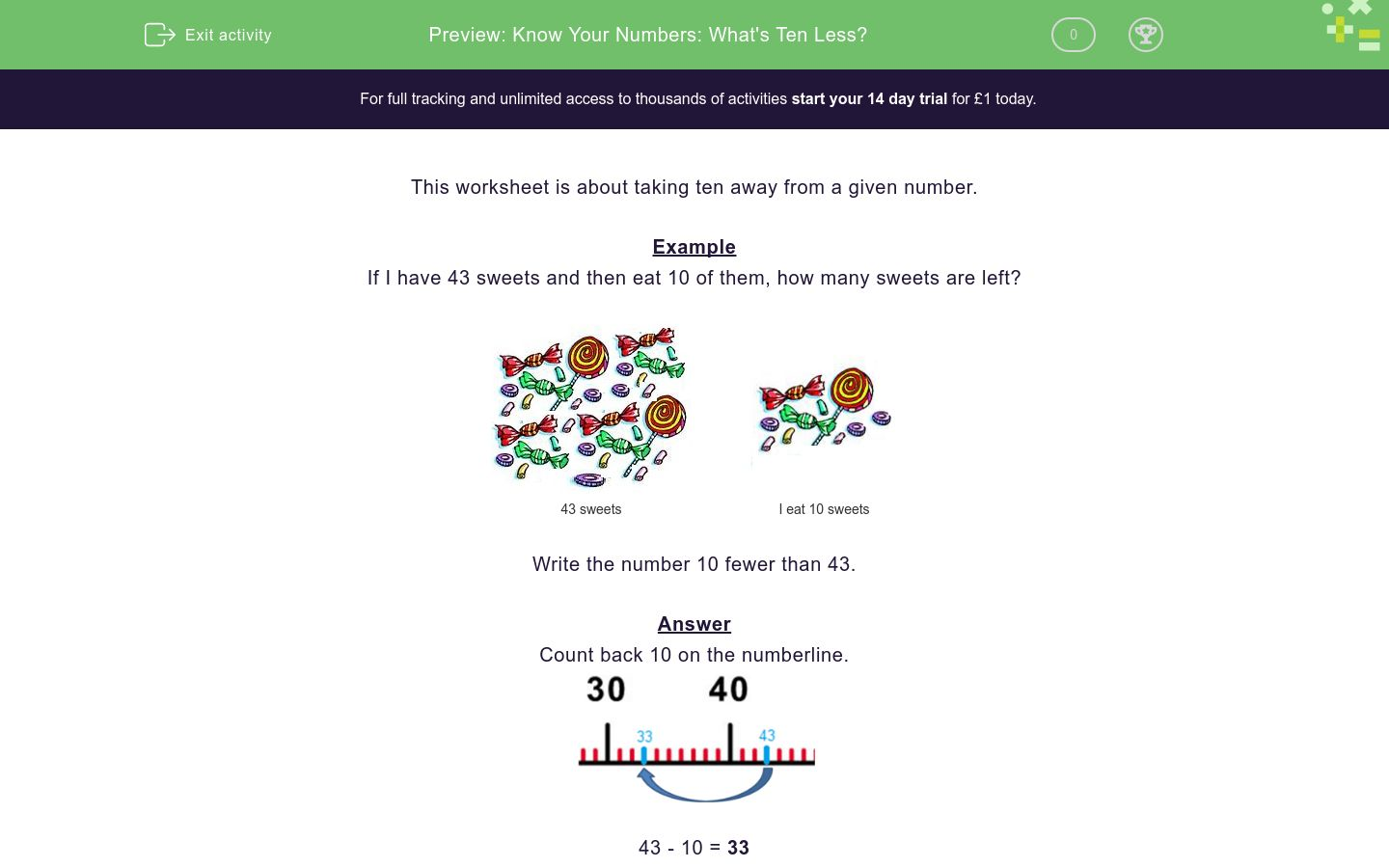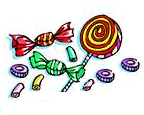# Know Your Numbers: What's Ten Less?

In this worksheet, students subtract 10 from a given number (in a range between 0 and 100).Key stage:  KS 1

Curriculum topic:   Number: Number and Place Value

Curriculum subtopic:   Count in Steps (2, 3, 5 and 10)

Difficulty level:### QUESTION 1 of 10

This worksheet is about taking ten away from a given number.

Example

If I have 43 sweets and then eat 10 of them, how many sweets are left?43 sweets I eat 10 sweets

Write the number 10 fewer than 43.

Count back 10 on the numberline.43 - 10 = 33

What is the number ten fewer than the given number?41 sweets I eat 10 sweetsWrite the number 10 fewer than 41.

What is the number ten fewer than the given number?33 sweets I eat 10 sweetsWrite the number 10 fewer than 33.

What is the number ten fewer than the given number?54 sweets I eat 10 sweetsWrite the number 10 fewer than 54.

What is the number ten fewer than the given number?48 sweets I eat 10 sweetsWrite the number 10 fewer than 48.

What is the number ten fewer than the given number?82 sweets I eat 10 sweetsWrite the number 10 fewer than 82.

What is the number ten fewer than the given number?87 sweets I eat 10 sweetsWrite the number 10 fewer than 87.

What is the number ten fewer than the given number?85 sweets I eat 10 sweetsWrite the number 10 fewer than 85.

What is the number ten fewer than the given number?50 sweets I eat 10 sweetsWrite the number 10 fewer than 50.

What is the number ten fewer than the given number?68 sweets I eat 10 sweetsWrite the number 10 fewer than 68.

What is the number ten fewer than the given number?78 sweets I eat 10 sweetsWrite the number 10 fewer than 78.

• Question 1

What is the number ten fewer than the given number?41 sweets I eat 10 sweetsWrite the number 10 fewer than 41.

31
EDDIE SAYS

41-10=31

• Question 2

What is the number ten fewer than the given number?33 sweets I eat 10 sweetsWrite the number 10 fewer than 33.

23
EDDIE SAYS

33-10=23

• Question 3

What is the number ten fewer than the given number?54 sweets I eat 10 sweetsWrite the number 10 fewer than 54.

44
EDDIE SAYS

54-10=44

• Question 4

What is the number ten fewer than the given number?48 sweets I eat 10 sweetsWrite the number 10 fewer than 48.

38
EDDIE SAYS

48-10=38

• Question 5

What is the number ten fewer than the given number?82 sweets I eat 10 sweetsWrite the number 10 fewer than 82.

72
EDDIE SAYS

82-10=72

• Question 6

What is the number ten fewer than the given number?87 sweets I eat 10 sweetsWrite the number 10 fewer than 87.

77
EDDIE SAYS

87-10=77

• Question 7

What is the number ten fewer than the given number?85 sweets I eat 10 sweetsWrite the number 10 fewer than 85.

75
EDDIE SAYS

85-10=75

• Question 8

What is the number ten fewer than the given number?50 sweets I eat 10 sweetsWrite the number 10 fewer than 50.

40
EDDIE SAYS

50-10=40

• Question 9

What is the number ten fewer than the given number?68 sweets I eat 10 sweetsWrite the number 10 fewer than 68.

58
EDDIE SAYS

68-10=58

• Question 10

What is the number ten fewer than the given number?78 sweets I eat 10 sweetsWrite the number 10 fewer than 78.

68
EDDIE SAYS

78-10=68

---- OR ----

Sign up for a £1 trial so you can track and measure your child's progress on this activity.

### What is EdPlace?

We're your National Curriculum aligned online education content provider helping each child succeed in English, maths and science from year 1 to GCSE. With an EdPlace account you’ll be able to track and measure progress, helping each child achieve their best. We build confidence and attainment by personalising each child’s learning at a level that suits them.

Get started